# What is the mathematical formula of electric charge

## Electric charge: examples, unit and calculate

Let's look at the electric charge here. Summary of the content:

• A Explanationwhat electrical charges are, what they are and how they behave.
• Lots Examples all about electrical charges.
• tasks / Exercises so that you can test your own knowledge on this topic.
• A Video, which deals with electrical charges.
• A Question and answer area to this topic.
Note:

### Explanation of electric charge

Let us first clarify the basics: What is an electrical charge and what electrical charges are there?

Note:

The electric charge is a fundamental property of matter. There are two types of electrical charge: there are positive electrical charges and negative electrical charges. Electrical phenomena are based on the accumulation or movement of charges.

Positive charges are marked with a plus (+) and negative charges are marked with a minus (-). These also show certain behaviors among each other, which we will look at in a moment.

We now take two charged spheres. One positively charged sphere and one negatively charged sphere. These two attract each other: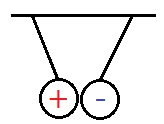Two positively charged spheres repel each other: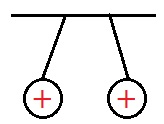Two negatively charged spheres also repel each other: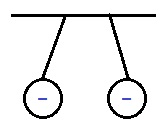Attract and repel:

• The same charges repel each other.
• Unequal charges attract each other.

In the subjects of chemistry and physics one deals with the structure of atoms again and again. Here the students are taught that there are protons in the nucleus of an atom and electrons in the shell. The protons are positive and the electrons are negative.

A body that has a negative effect on the outside has an excess of electrons. A body that has a positive effect on the outside, on the other hand, lacks electrons (lack of electrons). Of course there is also a third possibility: the body is electrically neutral. In this case there are the same number of protons and electrons. Let's look at this again with pictures.

A total of negatively charged body: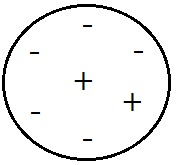A total of positively charged body: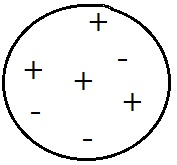Electrically neutral body: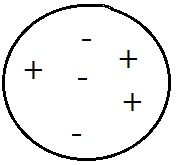The smallest possible electrical charge is called Elemental charge. Every electrical charge is a multiple of this elementary charge

Formula symbol and unit:

How heavy someone is is usually stated in kilograms. The height of a book is usually given in centimeters. But how do you measure the amount of charges? Of course, you don't do this in kilograms or centimeters, but in Coulomb. This is simply abbreviated with a C. The smallest possible charge is the elementary charge "e". It amounts to:

Formula charge body:

The charge on a body can be calculated using the following equation: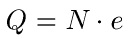The following applies:

• "Q" is the amount of charge in coulombs
• "N" is the number of charges
• "e" is the elementary charge of 1.602 x 10-19 C.

You can find an example with numbers below.

Display:

### Examples of electric charge

Examples of electrical charge are presented in this section. These examples correspond to what is often dealt with in school.

example 1:

Fill in the following blanks:

• Charges of the same name ___________ one to.
• Charges of the same name ___________ from each other.

Solution:

• Charges of a different name attract one.
• Charges of the same name repel each other.

Example 2:

This task is normally not dealt with in 5th grade, but only later. Nevertheless, it should be listed here for the sake of completeness. The problem: Let the number of charges be 6.24 · 1017. How big is the total charge?

Solution:

We use the formula to solve the problemWe also know how big the elementary charge e is: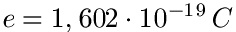The number of charges can be found in the task. So we just have to plug in and calculate Q.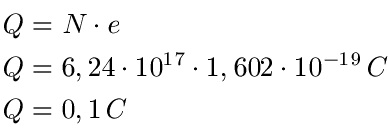The total charge is therefore 0.1 C.

Show:

### Video electric charge

The next video explains what the electric charge is all about. It deals with positive and negative charges. The relationship between electrons and protons is also shown. At the end of the video an example of charges is calculated.

Next video »

In this section we look at typical questions with answers about electrical charges.

Q: How can you detect electrical charges?

A: There are several possibilities and attempts to detect electrical charges:

• Electroscope: An electroscope is used to detect the electrical charge on a body. This is a measuring device with a pointer. The pointer deflection is a measure of the size of the charge.
• Ruler and cloth: You take a plastic ruler and hang it on a thread. Rub it vigorously with a cloth to charge it. This is exactly what you do with a second ruler. If you now bring both rulers together, they repel each other.

Q: What is Influence?

A: Influence is understood as the change in the charge distribution on a body when a charged body approaches. If we bring a charged body close to an electrically uncharged body, then the charged body exerts forces on the uncharged body, which lead to the movement of electrons.

Q: Can charges also be separated?

A: Yes, in principle charges can be separated by touching or rubbing. Electrons pass from one body to another. There is also a charge separation through electrochemical processes. This is used with accumulators and galvanic elements.

Q: What other topics should I check out?

A: For the basics of electrical engineering and magnetism, you should still have a look at these topics: